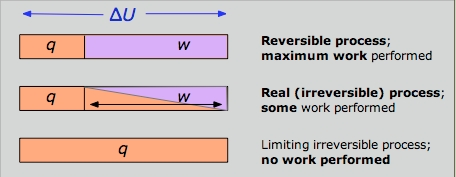## Free Energy and Work

#### Learning Objective

• Identify the relationship between Gibbs free energy and work.

#### Key Points

• When a system changes from a well-defined initial state to a well-defined final state, the Gibbs free energy, ΔG, equals the work done/exchanged by the system with its surroundings, minus the work of the pressure forces.
• “Useful” work is most commonly electrical work (moving electric charge through a potential difference).

#### Term

• workIn thermodynamics, work performed by a closed system is the energy transferred to another system that is measured by the external generalized mechanical constraints on the system.

## Gibb’s Free Energy and “Useful” Work

Gibbs energy is the maximum useful work that a system can do on its surroundings when the process occurring within the system is reversible at constant temperature and pressure. As in mechanics, where potential energy is defined as capacity to do work, different potentials have different meanings. The Gibbs free energy is the maximum amount of non-expansion work that can be extracted from a closed system. The work is done at the expense of the system’s internal energy. Energy that is not extracted as work is exchanged with the surroundings as heat.Work DiagramThe reversible condition implies wmax and qmin. The impossibility of extracting all of the internal energy as work is essentially a statement of the Second Law.

The appellation “free energy” for G has led to so much confusion that many scientists now refer to it simply as the “Gibbs energy. ” The “free” part of the older name reflects the steam-engine origins of thermodynamics, with its interest in converting heat into work. ΔG is the maximum amount of energy which can be “freed” from the system to perform useful work. “Useful” in this case, refers to the work not associated with the expansion of the system. This is most commonly electrical work (moving electric charge through a potential difference), but other forms of work are also possible. For instance, examples of useful, non-expansion work in biological organisms include muscle contraction and the transmission of nerve impulses.